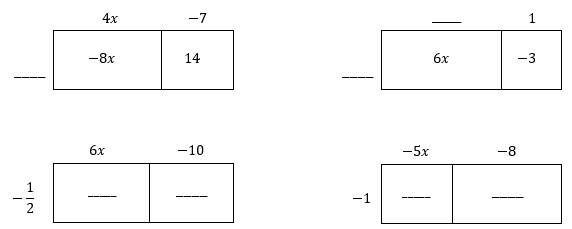# Numerical and Algebraic Expressions

## Objective

Expand and factor expressions with negative rational numbers.

## Common Core Standards

### Core Standards

?

• 7.EE.A.1 — Apply properties of operations as strategies to add, subtract, factor, and expand linear expressions with rational coefficients.

?

• 6.EE.A.3

• 7.NS.A.2

## Criteria for Success

?

1. Use the distributive property to expand an expression by distributing a negative term through a sum or difference in parentheses.
2. Model the distributive property with a negative factor using rectangular arrays.
3. Apply the rules of multiplying signed numbers to expanding and factoring expressions.

## Tips for Teachers

?

In Lessons 3 and 4, students expand and factor expressions with rational numbers. In Lesson 4, students see negative numbers both inside and outside a parentheses group. They will need to recall rules of multiplying signed numbers, specifically a negative times a negative is a positive number.

#### Fishtank Plus

• Problem Set
• Student Handout Editor
• Vocabulary Package

## Anchor Problems

?

### Problem 1

For each problem, fill in the blanks around the diagram, and then write equivalent expressions in the form:

___ ( _______) = ___________### Problem 2

What are the values of $q$ and $r$ in each set of equivalent expressions?

a.     $q(6x+4)=3x+r$

b.     $q\left ( \frac{1}{2}x+\frac{1}{3} \right ) = 3x+r$

c.     $q(3x+4)=r-8$

### Problem 3

Expand or factor each expression.

a.     ${-\frac{1}{2}(-5x+4y-16)}$

b.     ${-2\left ( 4-\frac{1}{2}h \right )}$

c.     ${-\left ( \frac{2}{3}a-\frac{3}{4}b-1 \right )}$

d.     ${-16xy+8y}$

e.     ${\frac{1}{2}x-1}$

## Problem Set

?The following resources include problems and activities aligned to the objective of the lesson that can be used to create your own problem set.

 Factored Form Expanded Form $-5(a-b+1)$ $18xy-6y$ $-\frac{2}{5}(-20x+10y)$ $-12d+9e-15$